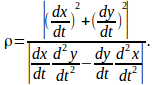## An Equation For Radius of Curvature For a Curve in the xy Plane

For a curve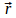in the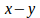plane, the radius of curvature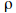is given by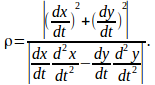To show this write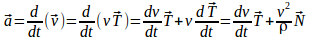where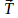and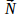are unit vectors. with find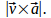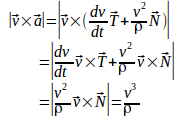since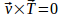and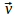is perpendicular to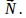Hence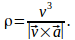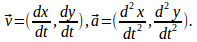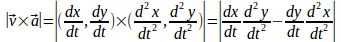Then